## # 第一章 绪论

### # 引言

#### # 语言

1. 字母表的定义
2. 词法规则单词符号的形成规则
3. 语法规则：（短语、从句、句子、段落、文章）语法单位的形成规则
4. 语义规则单词符号语法单位含义规则
5. 语用规则：语义规则的发展和延伸。在一定的语境中，单词和语法单位体现出来的具体意义，需要根据上下文进行明确

#### # 机器语言、汇编语言、高级语言

机器语言 -> 汇编语言 -> 高级语言

• 机器语言：由二进制指令组成。计算机可以直接执行
• 汇编语言：将机器语言符号化的结果
• 低级语言：与机器有关的语言（指令的操作码、指令格式、寻址方式、数据格式）
• 高级语言：和机器无关的语言

#### # 翻译

• 翻译：等价的变换

• 翻译过程：

• 实现：编写一个高级语言的编译程序的工作，通常称为对这个语言的实现

• 源语言、宿主语言、目标语言
• 源程序、编译程序、目标程序 高级语言涉及的三类人：
• 设计者、实现者、使用者

#### # 解释

• BASIC 是最简单的高级语言。BASIC 程序不是编译执行，而是对源程序进行解释（分析），直接计算出结果。
• 解释需要解释程序(解释器)
• LISP，ML，Prolog 和 Smalltalk 均是解释型的语言。
• Java 被当作一种解释型语言。翻译产生字节码的中间代码， 可以在 Java 虚拟机上运行。

• 解释执行特别适合于动态语言交互式环境，便于人机对话。
• 重复执行的语句需要重复翻译，比编译执行要花去更多的时间，执行效率较低。

### # 强制式语言、函数式语言、逻辑式语言、对象式语言

1. 强制式语言:基础是冯·诺依曼模型
2. 函数式语言:基础是数学函数(函数运算)
3. 逻辑式语言:基础是数理逻辑、谓词演算
4. 对象式语言:基础是抽象数据类型

#### # 绑定

1. 实体：程序的组成部分，如变量，表达式、程序单元等
2. 属性：实体具有的特性
3. 绑定：实体与其各种属性建立起联系的过程称为绑定（约束）
4. 描述符(表)：描述实体属性的表格

#### # 静态和动态

• 静态特性：编译时能确定的特性
• 动态特性：运行时才能确定的特性

• 静态绑定：绑定在编译时完成，且在运行时不会改变。
• 动态绑定：绑定在运行时完成。

• 静态作用域绑定:按照程序的结构定义变量的作用域（C语言等）。依据定义变量的位置进行确定。
• 动态作用域绑定:按照过程的调用关系动态地定义变量的作用域（SNOBL4 语言等）

#### # 变量的四个属性

1. 作用域：全局变量、局部变量、非局部变量
2. 生存期
3. 类型

## # 第四章 程序语言的设计

### # 前言

<标识符>→<字母>
<标识符>→<标识符><字母>
<标识符>→<标识符><数字>
<字母>→A|…|Z|a|…|z
<数字>→0|…|9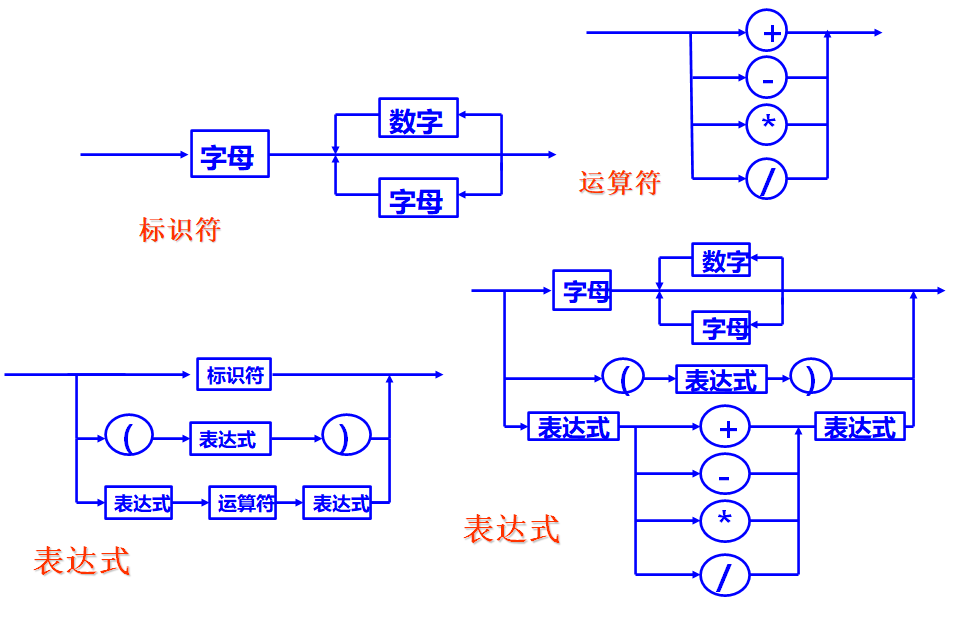### # 文法

#### # 文法的形式定义

• $V_T$终结符的非空有限集；
• $V_N$非终结符的非空有限集；
• $V=V_T \bigcup V_N$
• $S$ 是文法的开始符号，有 $S\in V_N$
• $P$ 为产生式 $\alpha\to\beta$ 的非空有限集，其中：
• $\alpha$$\beta$ 是由终结符、非终结符组成的串，且 $\alpha$ 至少应含有一个非终结符。即 $\alpha=V^*V_NV^*, \beta=V^*$
• $^*$ 表示前面的符号重复零次或多次（这很正则表达式）

G: Grammar
T: Terminal Symbols
N: Non-Terminal Symbols
S: Start Symbol
P: Production

#### # 产生式的简化

\left.\begin{aligned} \alpha&\to\beta_1 \\ \alpha&\to\beta_2 \\ &...\\ \alpha&\to\beta_n \end{aligned}\right\} \alpha\to\beta_1|\beta_2|\beta_n

$\beta_1, \beta_2, \beta_n$ 称为 $\alpha$ 的候选式。

#### # 文法的表示

\begin{aligned} E &→ E+T | E-T | T \\ T &→ T*F | T/F | F \\ F &→ (E) | i \end{aligned}

#### # 文法的分类

0型文法(PSG) $\alpha\to\beta$ 无限制
1型文法(上下文有关文法CSG) $\alpha$ 长度小于 $\beta$ 长度（$S→\varepsilon$例外） 右边的表达式长于左边
2型文法(上下文无关文法CFG) $A→\beta$ 左边是独立的非标识符
3型文法(正则文法RG,或右线性文法RLG) $A→u$$A→wB$, $u\in V_T^*, w\in V_T^+$ 右边不以非标识符开头

#### # 推导的表示

• 直接推导（由产生式右边替换产生式左边）： $w\alphaγ\Rightarrow w\betaγ$
• 任意步推导（0 步或多步）：$y \Rightarrow^* z$
• 多步推导（1 步或多步）：$y \Rightarrow^+ z$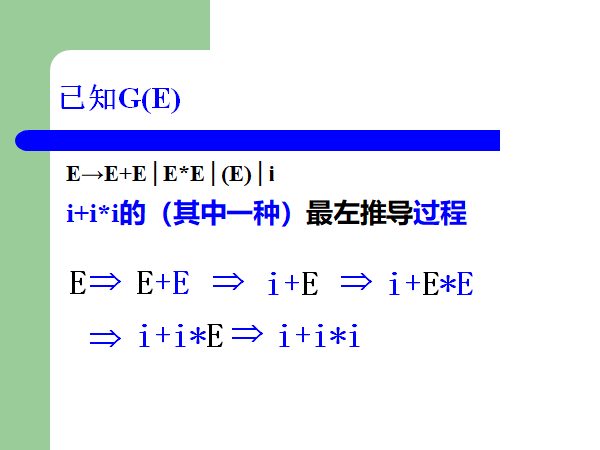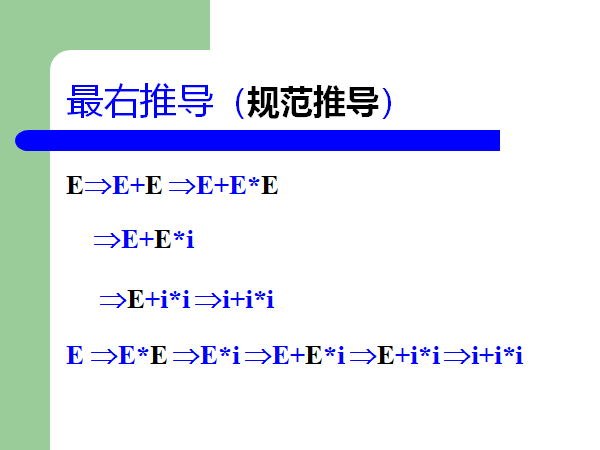#### # 句型、句子、语言

$G = (V_T, V_N, S, P)$

• 句型：S 能推导出的 $w$
• 句子：S 能推导出的只含终结符的 $w$
• 语言：G 产生的所有句子的集合 $L(G)=\{\alpha|S\Rightarrow^+\alpha$$\alpha\in V_T^*\}$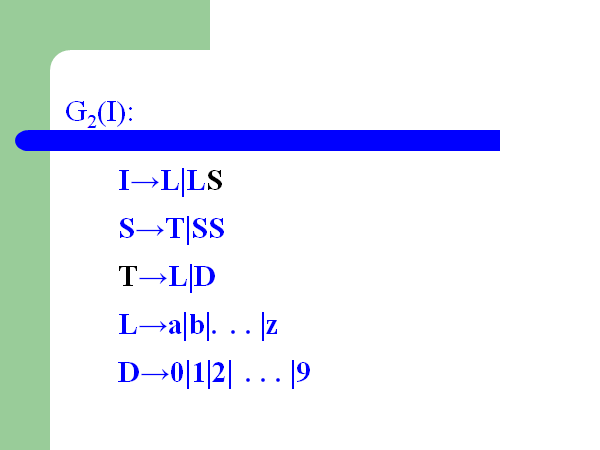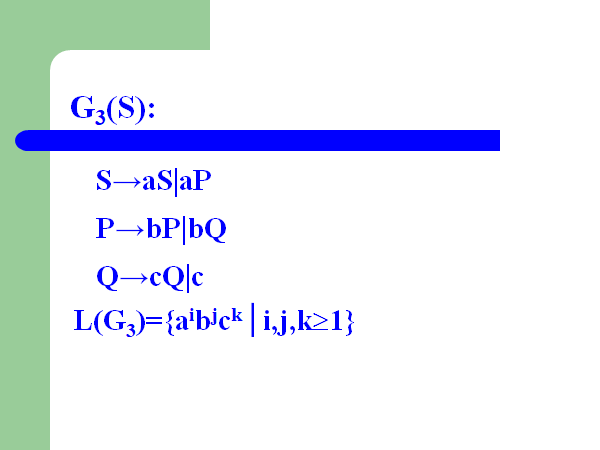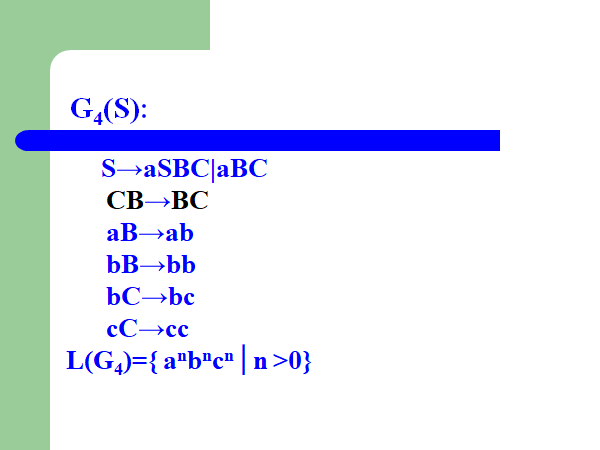#### # 推导树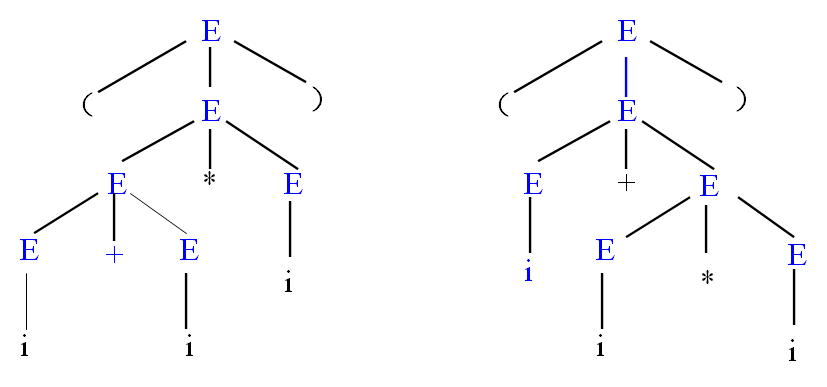## # 第五章 编译

### # 复习概念

• 源语言、宿主语言、目标语言
• 源程序、编译程序、目标程序

• 自驻留：若编译程序生成宿主机执行的机器代码，称为自驻留的编译程序
• 自编译：若编译程序用源语言编写的，则为自编译的编译程序
• 交叉编译：若编译程序生成不是宿主机(别的机器)执行的机器代码，称为交叉编译

### # 编译步骤

1. 源程序的分析
2. 目标程序的合成

1. 词法分析
2. 语法分析
3. 语义分析与中间代码生成
4. 中间代码优化
5. 目标代码生成

#### # 词法分析

• 分析输入字符串
• 根据词法规则识别出单词符号：基本字、标识符、字面常量、运算符和界符

#### # 语法分析

• 根据语法规则，识别各类语法单位：表达式、语句、程序单元和程序
• 进行语法检查

#### # 语义分析与中间代码生成

• 根据语义规则，对语法正确的语法单位进行翻译
• 可以直接生成目标程序
• 但目标程序的执行效率低
• 因此，生成中间代码

• 大多数编译器采用中间代码来描述源程序的语义。
• 这种中间语言对应某种抽象机
• 结构简单，语义明确，易于翻译成目标代码，同时也便于优化和移植。

#### # 优化

• 对中间代码进行等价变换，提高代码的时空效率。
• 语义分析产生的中间代码不依赖于实际机器，易于实现一些等价变换，使生成的目标程序占用空间更少，执行更快。

#### # 目标代码生成

• 根据优化后的中间代码及有关信息，可生成较为有效的目标代码：
• 目标机的机器语言程序或汇编语言程序
• 若生成的是汇编语言程序，还需由将其汇编成机器语言程序。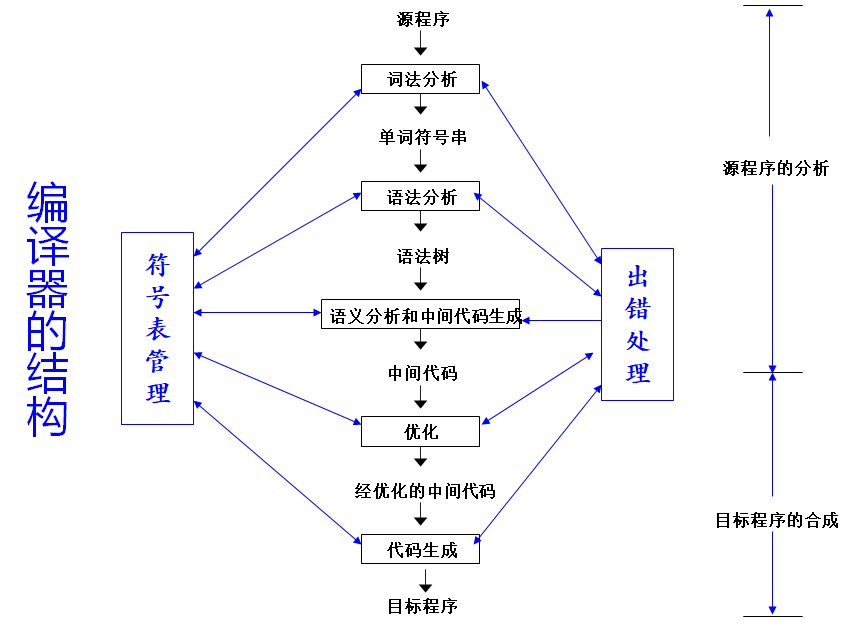### # 完整的程序处理过程

1. 预处理器
2. 汇编器
3. 连接器
4. 装入器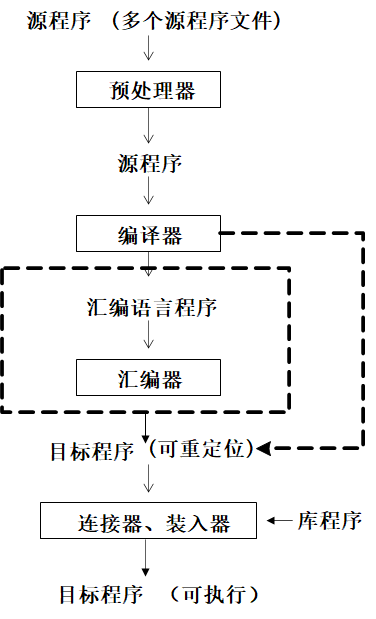### # 编译前端和后端

• 在现代编译器中，通常将编译过程划分为前端和后端两大部分，分别加以实现。
• 前端和后端通过中间代码连接，可极大的提高编译器设计与实现的效率。
• 前端：主要是与源程序相关的部分，包括词法、语法分析、语义分析和中间代码生成等。
• 后端：主要是与目标程序相关的部分，包括优化、目标代码生成等。

## # 第六章 词法分析

### # 概述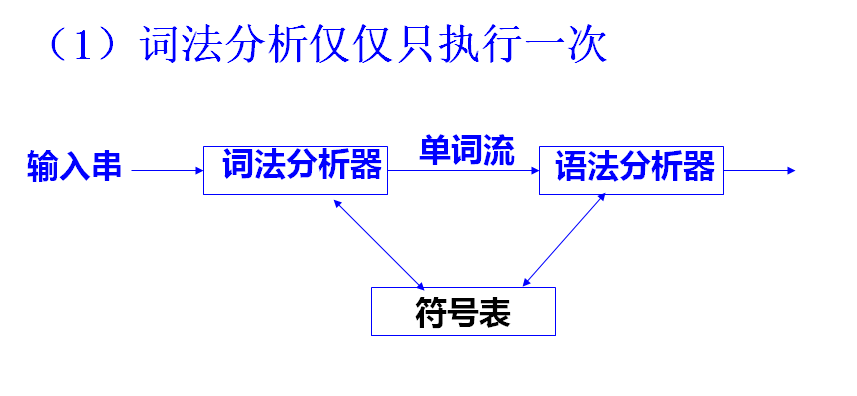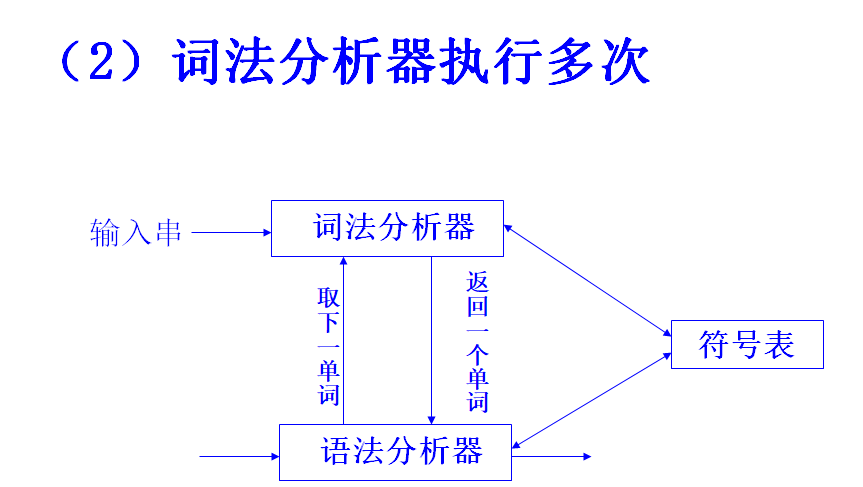### # 单词的种类

• 标识符
• 关键字
• 常量
• 运算符
• 分界符
while(*pointer!='\0') pointer++;

while       关键字
(           界符
*           运算符
pointer     标识符
!=          运算符
'\0'        常量
)	        界符
pointer     标识符
++          运算符
;           界符
'\n'        界符


### # 单词的输出形式

• 单词类别：区分单词所属的类(整数编码)
• 单词：单词的属性值

### # 单词类别的划分

• 标识符通归一类。
• 常量可按整型、实型、字符型、布尔型等分类。
• 常量或标识符将由第二元----单词的属性值来区别：
• 通常将常数在常量表中的位置（编号）作为常量的属性值；
• 将标识符在符号表中的位置（编号）作为标识符的属性值。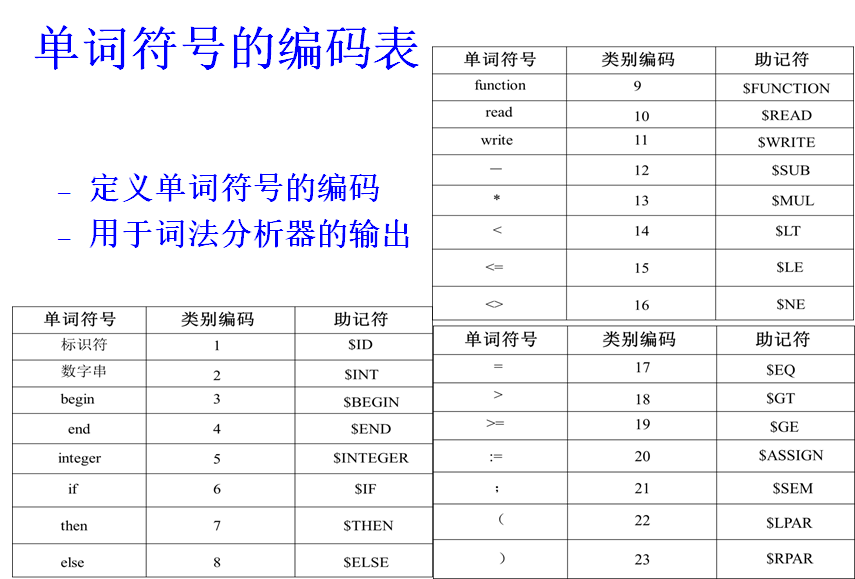### # 状态转换图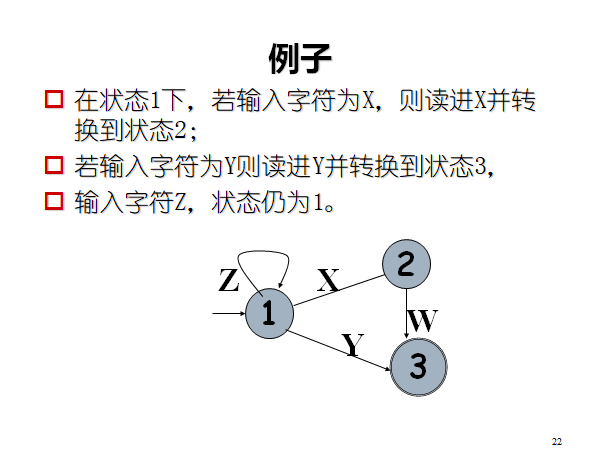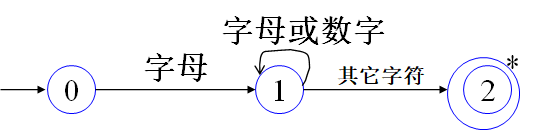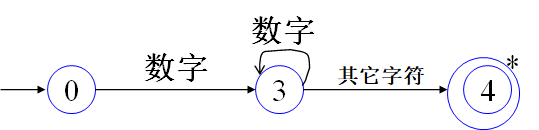### # 词法分析器的转换图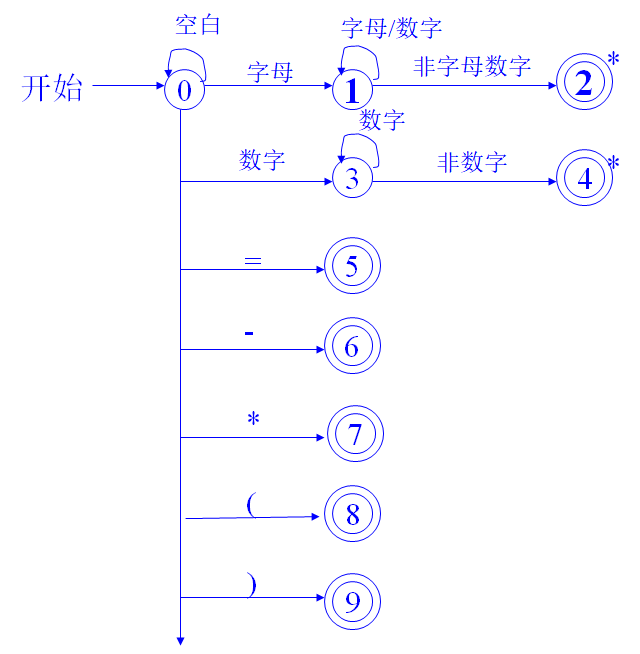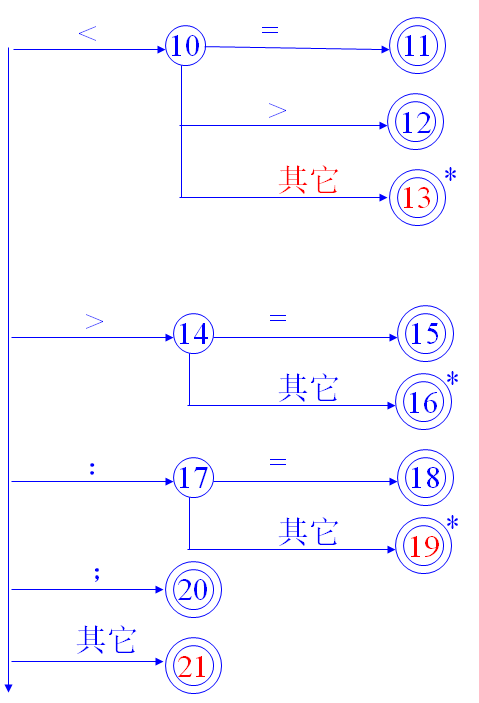## # 第七章 自上而下的语法分析

• 编译理论中，语法分析是对高级语言的语法单位的结构进行分析。
• 语法单位结构可以用上下文无关文法来描述。
• 下推自动机可用于识别上下文无关文法所描述的语法单位。
• 上下文无关文法及下推自动机是语法分析的理论基础。

### # 引言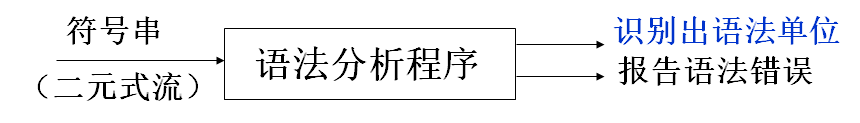• 自上而下(自顶向下)的分析方法（第七章）：从 $S$ 出发，能否找到一个最左推导序列，使得 $S\Rightarrow^*w$；或者从根结点 $S$ 开始，能否构造一棵语法树，使得该语法树的叶结点自左至右的连接正好是 $w$
• 自下而上(自底向上)的分析方法（第八章）：从 $w$ 出发，能否找到一个最左规约（最右推导的逆过程）序列，逐步进行规约，直至文法的开始符号 $S$

• 回溯分析法是不确定的分析方法。
• 递归下降分析法预测分析法属于确定的分析方法。

### # 回溯分析法

\begin{aligned} S&→xAy \\ A&→ab|a \end{aligned}

• 采取试探方法来分析每一个候选式，分析的过程很可能产生回溯
• 可能产生回溯的原因有： (1)公共左因子 (2)左递归 (3)$\varepsilon$ 产生式

#### # 公共左因子

$A→\alpha\beta_1|\alpha\beta_2$
• 若所有候选式都没有公共左因子，就可以选择惟一匹配的候选式，减少不必要的回溯

#### # 左递归

• 左递归：$A\Rightarrow^+A\beta$
• 直接左递归：$A\Rightarrow A\beta$

#### # 主要缺陷

1. 选择候选式进行推导是盲目
2. 若文法存在左递归，语法分析还可能产生无限递归
3. 引起时间和空间的大量消耗
4. 无法指出语法错误的确切位置

• 要么只有一个候选式可用
• 要么没有候选式可用

### # 递归下降分析法

#### # 方法步骤

1. 提取公共左因子（看 PPT）
2. 消除左递归
• 消除直接左递归（改写为右递归）
• 间接左递归（$A\Rightarrow^+A\alpha$）的消除利用算法进行 a. 将文法 $G$ 的所有非终结符按任一给定的顺序排列为 $A_1, A_2, ..., A_n$ b. 消除可能的左递归（算法见后） c. 化简：删除多余产生式
// 消除左递归
for i:=1 to n do
for j:=1 to i-1 do
把形如Ai→Ajα的产生式改写为
Ai→δ1α|δ2α|...|δkα
消除Ai产生式可能的直接左递归


\begin{aligned} S&→Qc|c \\ Q&→Rb|b \\ R&→Sa|a \\ \end{aligned}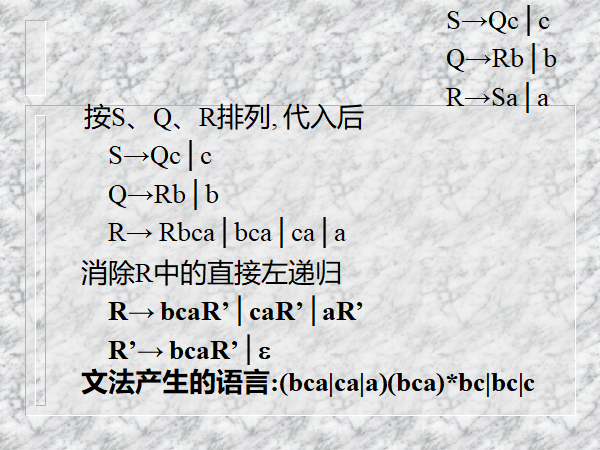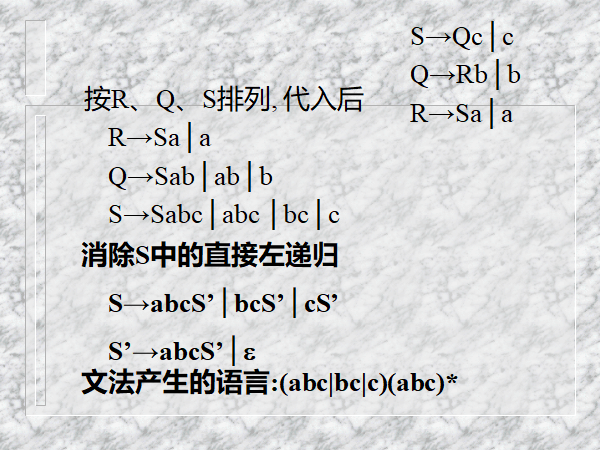#### # 递归下降分析器的构造

• 没有任何公共左因子
• 没有任何左递归 则有可能构造出不带回溯的分析程序

### # 预测分析法

#### # 构造 First 集

1. A -> aB，A 就有 {a}
2. A -> B，A 可以把 B 的复制过来
3. A -> BC，若 B 可以为 \varepsilon，A 就还能复制 C 的

1. S

FIRSTVT E + ( * i T * ( i F ( i

## # 第八章 自下而上语法分析

### # 概念

• 短语：某个祖先的所有没孩子的子孙组成的语句
• 直接短语：某个没有孙子的爸爸的孩子组成的语句
• 句柄：最左直接短语
• 素短语：短语至少有一个终结符，且不不含更小的素短语（若 i 是素短语，则 Fi* 不是素短语）

### # 算符优先分析法

#### # 构造 FIRSTVT 集

1. A -> aBcA -> Bac，A 就有 {a}
2. A -> BC，A 可以把 B 的复制过来
3. A -> BC，若 B 可以为 \varepsilon，A 就还能复制 C 的

#### # 构造 LASTVT 集

1. A -> caBA -> cBa，A 就有 {a}
2. A -> BC，A 可以把 C 的复制过来
3. A -> BC，若 C 可以为 \varepsilon，A 就还能复制 B 的

#### # 算符优先关系表

1. 优先：先归约的符号被称为优先级高。
2. 先看行，再看列。如 +i 列为 >，则 + > i
3. 规则：若 E+T，则 LASTVT(E) > ++ < FIRSTVT(T)
• 还要添加 #S# 这个文法
• 这里的大于、小于没有对称性（a>b 不能推出 b<a
• 推荐先填等号，再按行填小于，再按列填大于

## # 第九章 语义分析

### # 符号表

名字 | 信息


### # 语义分析概论

#### # 语义分析的主要工作

(1) 类型检查：数据的使用应与类型匹配
(2) 控制流检查：用以保证控制语句有合法的转向点:

(3) 一致性检查：如数组维数是否正确; 变量是否已经定义；变量是否重名；case常量不能相同等。

#### # 语法制导翻译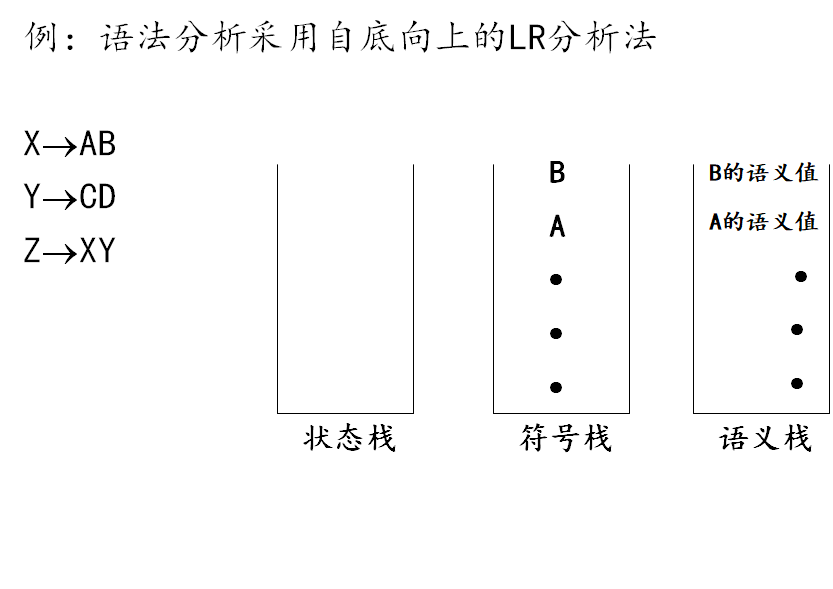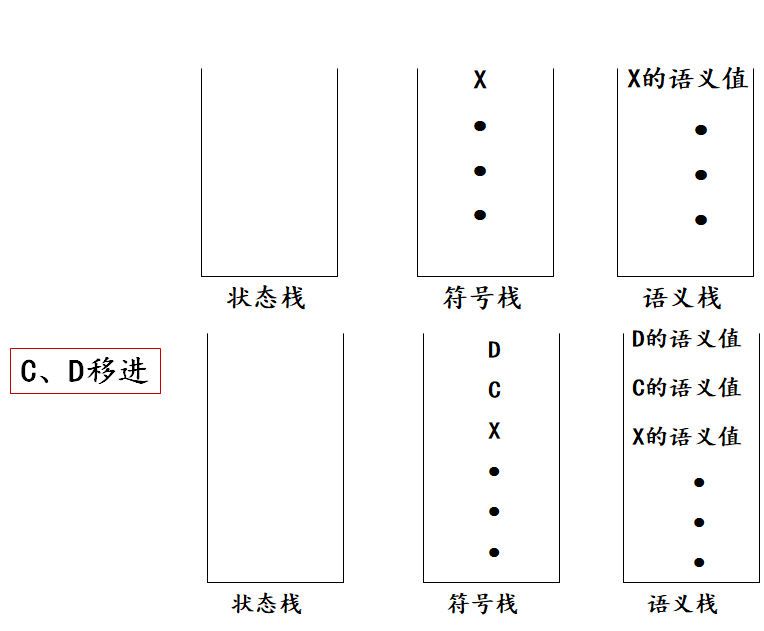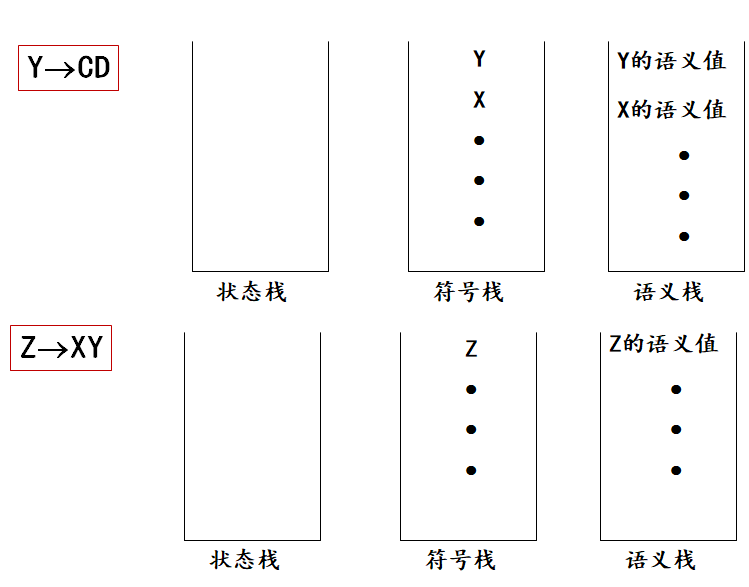#### # 语义值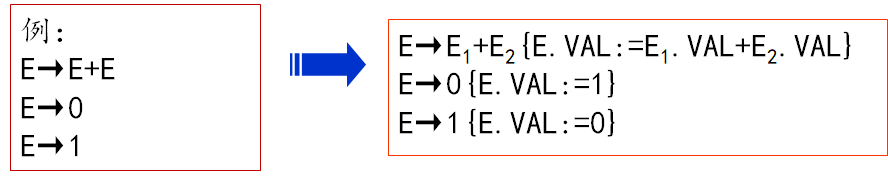#### # 中间代码

op—运算符
ARG1—第一运算量
ARG2—第二运算量
RESULT—结果

(@,B,_,t1)
(+,C,D,t2)
(*,t1,t2,t3)
(:=,t3,_,A)


### # 简单赋值语法分析的翻译

#### # 语义变量及过程

• X.a：文法符X相应属性a,如i.name,E.place。E.place:表示E所代表的变量在符号表的入口地址。
• newtemp：语义函数，每调用一次产生一个新的临时变量。
• entry(i)：语义函数，查符号表，返回变量i的入口。
• IP：指令指针，初始化为0，也可以是指定的初值。
• gen(OP,ARG1,ARG2,RESULT)：语义过程，生成一个四元式(OP,ARG1,ARG2,RESULT),并填入四元式表中。同时 ip:=ip+1

#### # 翻译方案

A → i:=E
E → E1 op E2 | -E1 |(E1)| i

A→i:=E
{
P=entry(i.NAME);
If(P!=0)
gen ( :=, E.place, _, P)
Else error();
}

E→E1 op E2
{
E.place:=newtemp;
gen(op,E1.place,E2.place,E.place)
}

E →-E1
{
E.place:=newtemp;
gen(@,E1.place,_,E.place)
}

E →(E1)
{  E.place:= E1.place  }

E →i
{  P=entry(i.NAME);
If(P!=0)  E.place:=P
Else error();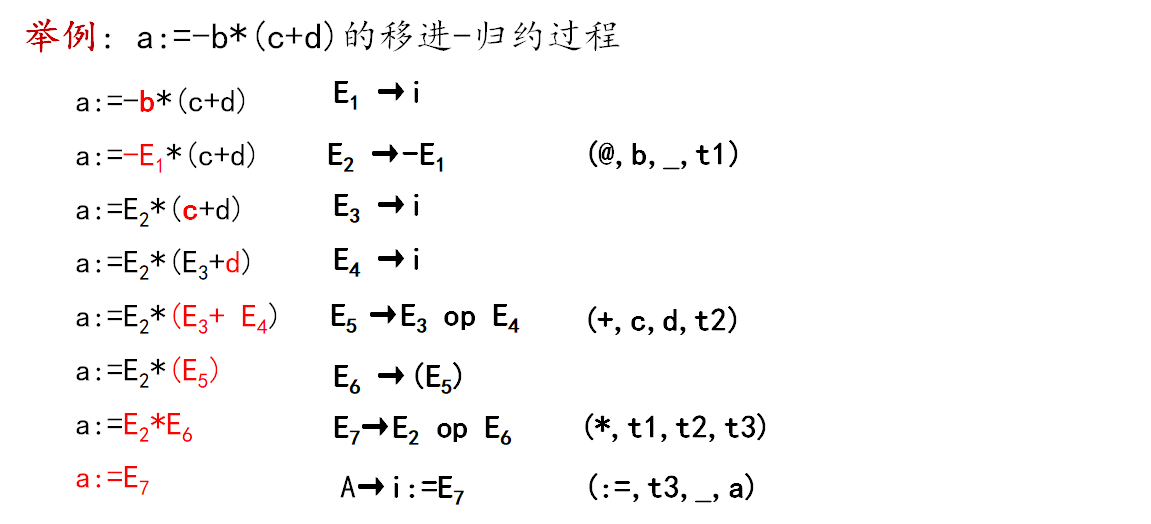#### # 类型转换

t:=newtemp;
if E1.type=integer
and E2.type=integer
then begin
gen(opi,E1.place,E2,place,t);
E.type:=integer
end

else if E1.type=real and E2.type=real
then begin
gen(opr,E1.place,E2.place,t);
E.type:=real
end

else if E1.type=integer then
begin t1:=newtemp;
gen(itr,E1.place,_,t1);
gen(opr,t1,E2.place,t);
E.type:=real
end

else begin t1:=newtemp;
gen(itr,E2.place,_,t1);
gen(opr,E1.place,t1,t);
E.type:=real
end;

E.place:=t;


### # 说明语句的翻译

#### # 文法

D→D;D|i:T
T→real|integer|array[num] of T1|↑T1


#### # 语义变量和过程

(1)offset:相对位移量,初值为0,是一个全局变量 (2)T.type:数据类型 (3)T.width:数据宽度 (4)enter:语义过程,将变量名及其类型和相对存储位置记入符号表中。

#### # 翻译方案

S →MD
M →\varepsilon        { offset:=0 }
D → D1;D2
D →i:T      { enter(i.name,T.type,offset); offset := offset+T.width }
T→integer {  T.type:=integer;  T.width:=4  }


### # 布尔表达式的翻译

#### # 文法

B→i
B→i1 rop i2

（1）if  B  then  S
（2）if  B  then  S else S
（3）while B do S


#### # 语义变量

• B.T：真出口，记录B为真的转移语句的地址；
• B.F：假出口，记录B为假的转移语句的地址。

#### # 转移语句的四元式

(jrop,P1,P2,0)
(jnz,P1,-,0)
(j,-,-,0)


0 是转移地址，从上往下分析的时候可能不知道要转义到哪里，所以会挖坑，后面会填坑。

#### # 翻译方案

B→i
{
B.T:=ip;
gen(jnz,entry(i),-,0);
B.F:=ip;
gen(j,-,-,0)
}

B→i1 rop i2
{
B.T:=ip;
gen(jrop,entry(i1),entry(i2),0);
B.F:=ip;
gen(j,-,-,0)
}


### # 无条件转移语句翻译

#### # goto L，L 已经定义

…
L: ...   /*此时，将L登记入符号表*/
…
goto L;  /*查表，发现L已定义，生成四元式*/


#### # goto L，L 未定义

…
goto L; /*将L记入符号表，定义否标记为“否”，
地址栏写当前IP，生产无条件转移 */
…
goto L; /*拉链，即生成向上指的链表*/
…
L: …   /*定义否标记改为“是”，回填，即根据链表依次改写跳转的地址栏*/
…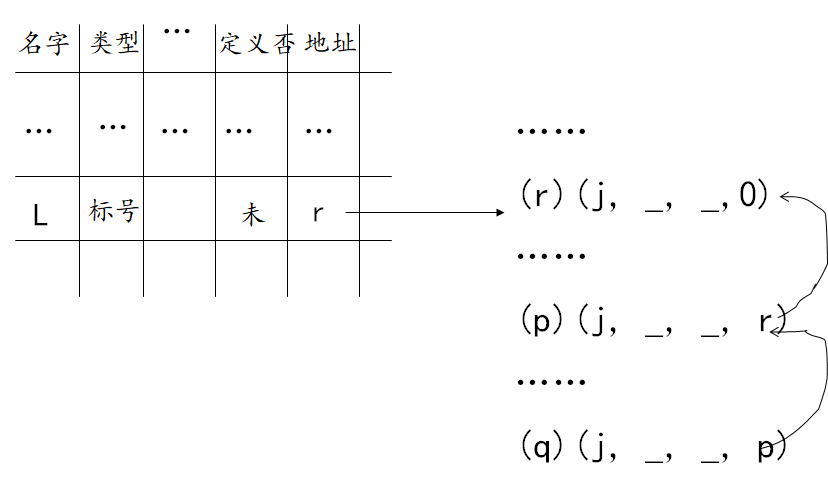### # 标号语句的处理方法

label →i:

1. i（假定为L）不在符号表中：则把它填入，置“类型”栏为“标号”，“定义否”栏为“已”，“地址”栏为ip的当前值；
2. 若L已在符号表中，“类型” 为“标号”, “定义否”栏为“否” :把“定义否”改为“已”，然后把“地址栏”中的链首q取出，同时把ip当前值填入其中，最后执行 backpatch（q，ip）语义过程进行回填
3. 若L已在符号表中，但“类型”不为“标号”,或“定义否” 为“已”：则“名字重定义”

backpatch（q，ip）为语义过程：把q为链首的链上所有四元式的第四区段都填为ip

### # If 条件语句的翻译

#### # 文法

S→if B then S1 | if B then S1 else S2


if B then S1：S1的第一条四元式 用以“回填” if B then S1 else S2：S1、S2的第一条四元式用以“回填”

(1)B具有真假出口 B为真假时的转向不同 在翻译B时其真假出口有待“回填” (2)因if语句的嵌套,必须记录不确定转移目标的四元式的地址—拉链技术

#### # 语义变量及过程

1. N.CHAIN：记录不确定转移目标的四元式形成的，不确定 label 地址时就调用这个一般来说，N.CHAIN 的含义是 N 结束以后要跳的位置
2. merge(t1,t2): 语义函数，合并链表，返回合并后的链头t2，用于合并链表/拉链
3. backpatch(t1,code): 语义过程，用code回填t1，确定 label 地址时就调用这个

(p) (j, -, -, 0)     (u) (j, -, -, 0)
……                     ……
(q) (j, -, -, p)     (v) (j, -, -, u)
……                     ……
(r) (j, -, -, q)     (w) (j, -, -, v)
t1=r                   t2=w


(p) (j, -, -, 0)    (u) (j, -, -, r)
……                 ……
(q) (j, -, -, p)    (v) (j, -, -, u)
……                 ……
(r) (j, -, -, q)    (w) (j, -, -, v)



(p) (j, -, -, 120)  (u) (j, -, -, 120)
……                   ……
(q) (j, -, -, 120)  (v) (j, -, -, 120)
……                   ……
(r) (j, -, -, 120)  (w) (j, -, -, 120)


#### # 翻译方案

if-then 不负责生成四元式，只负责填 0！！！

S→if B then S1
S→M S1
M→if B then

M→if B then
{
backpatch(B.T, ip); // B.T 即为下一句的地址，所以可以直接填当前 ip
M.CHAIN:=B.F        // B.F 在后面，所以创建链表
}

/*
* 另外,在图9-3中，整个语句翻译完成后,B.F仍不能确定,只能
* 将它作为S的语义值S. CHAIN暂时保留下来。如果S,本身也是控
* 制语句(比如另一个嵌套的条件语句),它也有语义值S. CHAIN未
* 确定,则B.F和S.CHAIN应转到同一个地方,因此要将它们链
* 接起来,组成一个新的链,链首地址记录在S的语义值S. CHAIN
* 中,这项工作由语义函数merge()完成。
*/
S→M S1
{
S.CHAIN:=merge(M.CHAIN, S1.CHAIN)   // B.F
}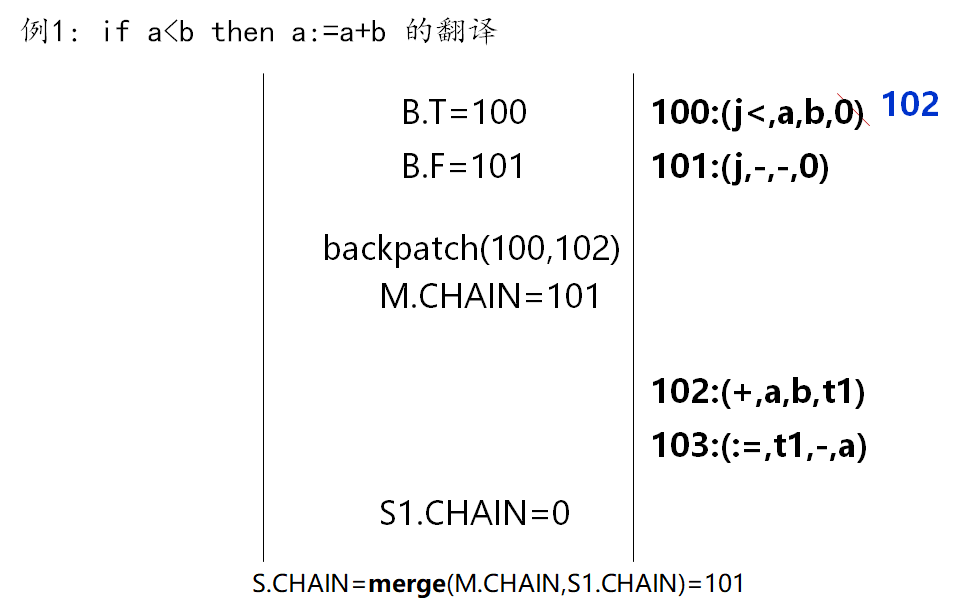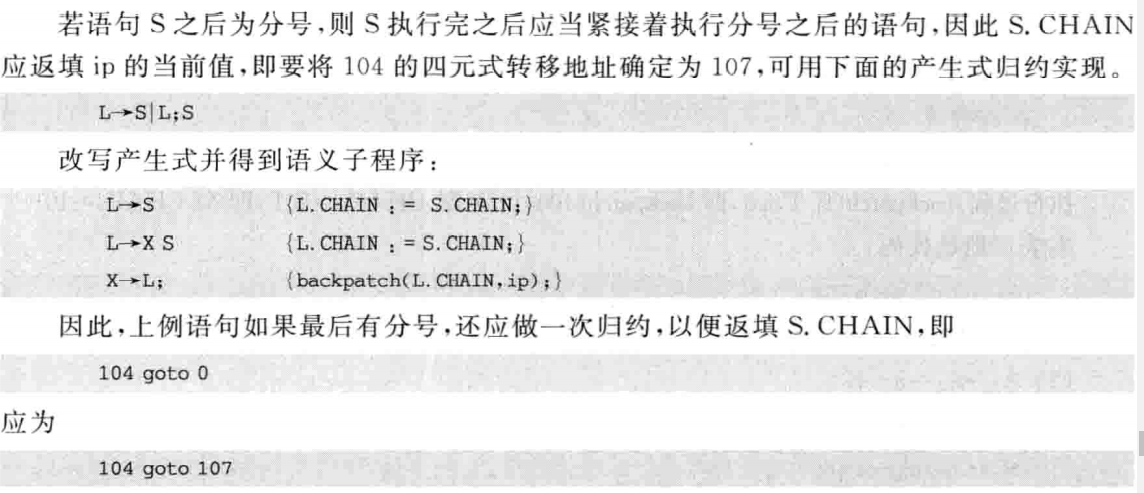if-then-else 只需要生成一个四元式：then 后的语句执行完以后要 jump 到 else 后

S→if B then S1 else S2
M→if B then
N→M S1 else
S→N S2

M→if B then
{
backpatch(B.T, ip);
M.CHAIN:=B.F
}

N→M S1 else
{
q:=ip;
gen(j,-,-,0);
backpatch(M.CHAIN,ip);
N.CHAIN:=merge(S1.CHAIN , q)
}

S→N S2
{
S.CHAIN:=merge(N.CHAIN, S2.CHAIN)
}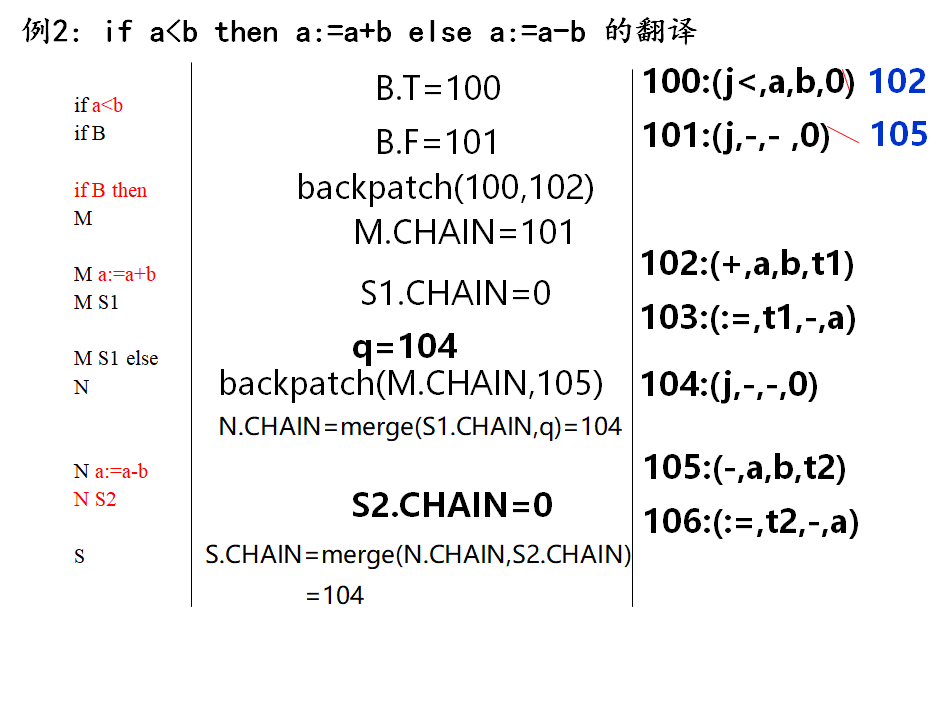### # While 语句的翻译

#### # 文法

S→while B do S1


#### # 翻译方案

while 语句其实就是 if-then 语句多了一个 jump

W→while
D→W B do
S→D S1

W→while
{
W.code := ip;
}

D→W B do
{
backpatch(B.T, ip);
D.CHAIN = B.F;
D.code = W.code;    // 子变量值传给父变量
}

S→D S1
{
backpatch(S1.CHAIN,D.code);
gen(j,-,-,D.code);
S.CHAIN:=D.CHAIN;
}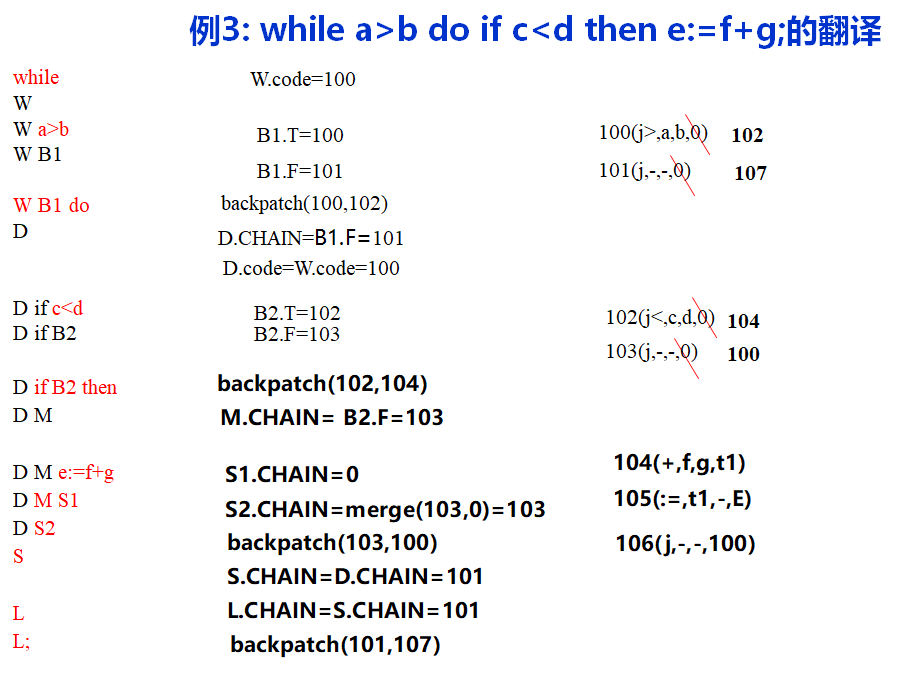S->A
{S.CHAIN=0;}


### # For 循环语句的翻译

#### # 文法

S→for i:=1 to N do S1


        i:=1;
again:  if i<=N then begin
S1;
i:=i+1;
goto again
end


F+0:（:=，'1'，-，i）
F+1: (j<=,i,N,F+3)
F+2: (j,-,-,0)
F+3: (S1的四元式序列)
...
D+0: (+,i, '1',i)
D+1: (j,-,-,F+1)


#### # 语义变量

F→for i:=1 to N do
S→F S1

• F.again:记录F+1
• F.CHAIN:记录前述F+2的地址
• F.place:记录i在符号表入口

#### # 翻译方案

F→for i:=1 to N do
{
gen(:=,'1',-,entry(i));
F.again:=ip;
gen(j<=,entry(i),N,F.again+2);
F.CHAIN:=ip;
gen(j,-,-,0);
F.place:=entry(i)
}

S→F S1
{
backpatch(S1.CHAIN,ip);
gen(+,F.place,'1',F.place);
gen(j,-,-,F.again);
S.CHAIN:=F.CHAIN
}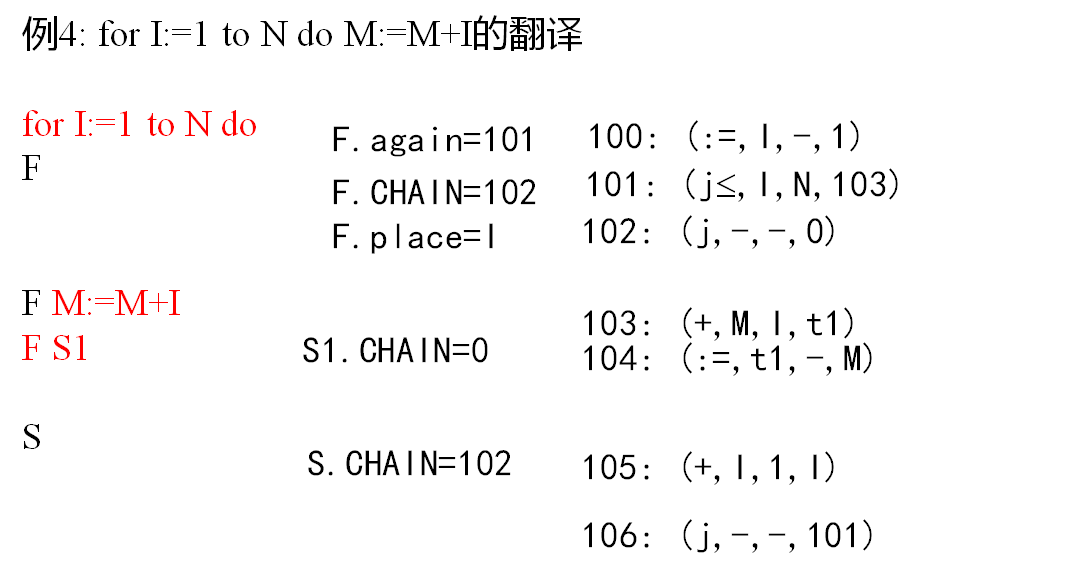for i=1 to 10 do if A>100 then C=C+1;

100: (=, 1, -, i)
101: (J<=, i, 10, 103)    F.again=103
102: (J, -, -, 109)
103: (J>, A, 100, 105)
104: (J, -, -, 107)
105: (+, C, 1, t1)
106: (=, t1, -, C)
107: (+, i, 1, i)
108: (J, -, -, 103)
109:


if  (A<X) & (B>0) then while C>0 do C:=C+D;


100 (j<， A， X， 102)
101 (j， -， -，  109)
102 (j>， B， 0， 104)
103 (j， -， -，  109)
104 (j>， C， 0， 106)
105 (j， -， -，  109)
106 (+， C， D，   T1)
107 (:=， T1， -， C)
108 (j， -， -，  104)
109


z := 3;
while j< 10 do
begin
j := x+1;
if x <10 then
y:= A+m;
else
y:= A-m;
end

100 (=, 3, _, z)
101 (J<, j, 10, 103)
102 (J, -, -, 113)
103 (+, x, 1, t1)
104 (=, t1, -, j)
105 (J<, x, 10, 107)
106 (J, -, -, 110)
107 (+, A, m, t2)
108 (=, t2, -, y)
109 (J, -, -, 112)
110 (-, A, m, t3)
111 (=, t3, -, y)
112 (J, -, -, 101)


## # 第十章 代码优化和目标代码生成

1. 源程序阶段的优化：优化数据结构和算法
2. 编译优化：中间代码和目标代码优化

1. 局部优化：在基本块内的优化
2. 全局优化：超越基本块，基本块之间的优化

### # 局部优化

#### # 划分基本块

(1)寻找入口语句

• 程序的第一条语句
• 由转向语句转移到的语句
• 紧跟在条件转向语句后的那个语句 (2)寻找出口语句
• 转向语句
• 停止语句 (3)基本块为：
• 每个入口地址（含）到下一个入口地址（不含）
• 每个入口地址（含）到下一个出口地址（含） (4)不在任何基本块中的代码可以被删除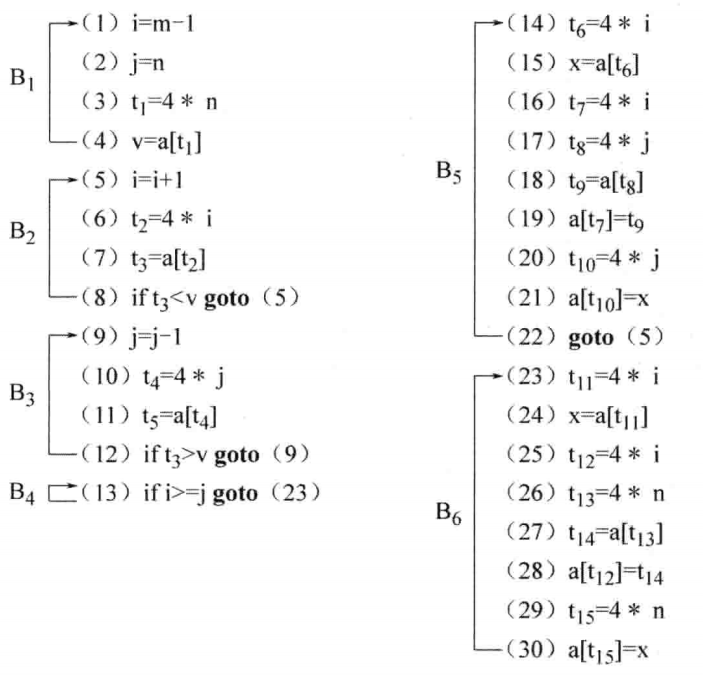#### # 构造流图

$G = (N, E, n_0)$
(1)

(2)包含程序第一个语句的基本块，为首结点n0; (3)设基本块 Bi , Bj ∈ N ，若满足下列条件之一，则Bi ->Bj

• Bj 紧跟在 Bi 之后，且 Bi 的出口语句不是无条件转向或停止语句
• Bi 的出口语句为转向语句，其转向点恰为Bj 的入口语句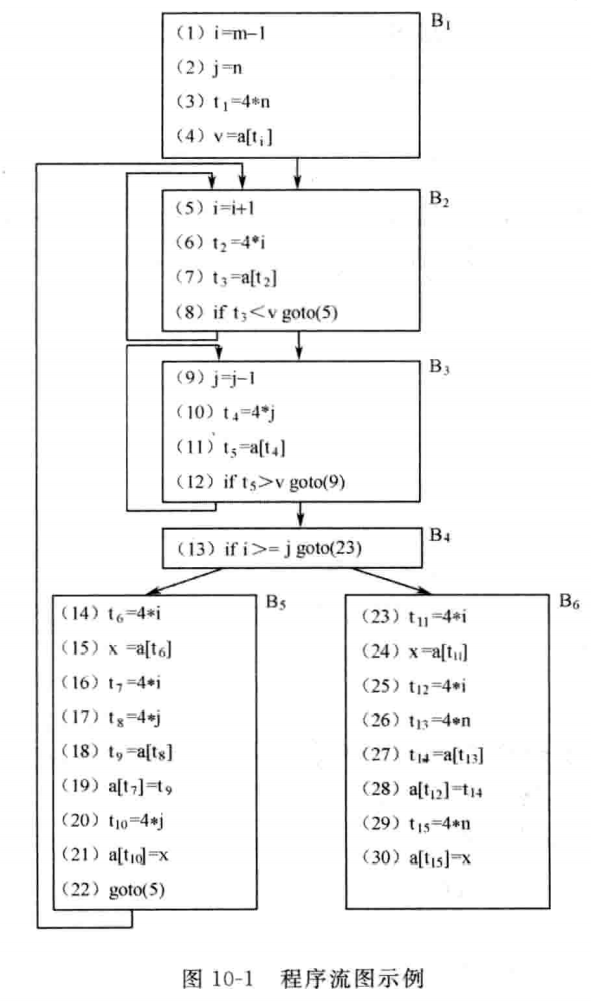#### # 基本块内的优化

##### # 合并已知量
// 优化前
B := 1
C := 2
A := B + C

// 优化后
A := 3

##### # 删除公共子表达式
// 优化前
B := 1
C := 2
A := B + C
D := B + C

// 优化后
D := A

##### # 删除无用赋值
// 优化前
A := 1
A := 2

// 优化后
A :=2


##### # 例题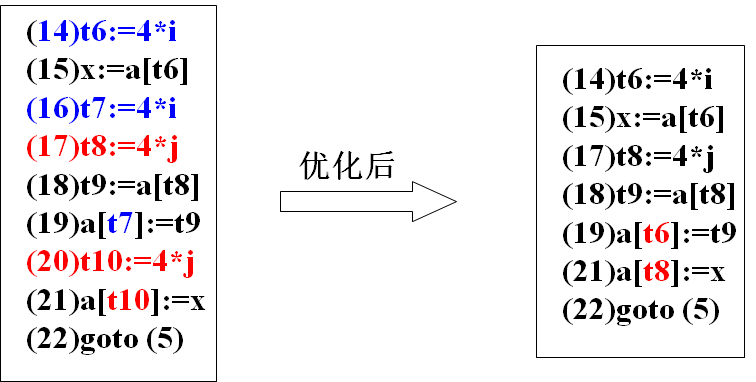### # 全局优化（自学）

#### # 循环的定义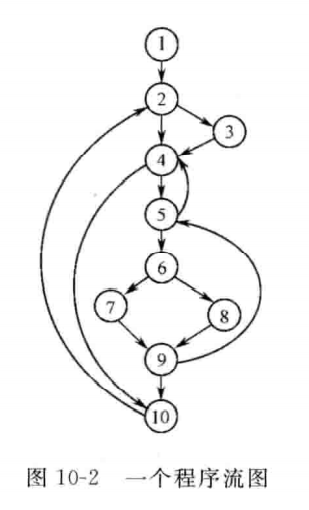{5, 6, 7, 8, 9} 是循环：所有点都互通，且 5 是唯一入口。

#### # 循环的查找

1. 必经结点：从流图的首结点出发，到达结点n的任一通路都必须经过的结点d，称为n的必经结点。记为 d DOM n
• 每个结点是它本身的必经结点，即n DOM n
• 首结点是任一结点的必经结点，即n0 DOM n

D(1)={1}
D(2)={1,2}
D(3)={1,2,3}
D(4)={1,2,4}
D(5)={1,2,4,5}
D(6)={1,2,4,5,6}
D(7)={1,2,4,5,6,7}
D(8)={1,2,4,5,6,8}
D(9)={1,2,4,5,6,9}
D(10)={1,2,4,10}

1. 回边：若 d 是 n 必经节点，且存在边 n->d，则该边为回边。

5->4
9->5
10->2

1. 若 n->d 是回边，则图里能到n且不经过d的点的集合+n+d 就是由 n->d 组成的循环5->4 组成 {5, 4, 6, 7, 8, 9}
9->5 组成 {9, 5, 6, 7, 8}
10->2 组成 {10, 2, 3, 4, 5, 6, 7, 8, 9}


#### # 循环优化方法

##### # 代码外提
// 优化前
for (int i = 0; i < n; i++)
{
float pi = acos(-1);
// ...
}

// 优化后
float pi = acos(-1);
for (int i = 0; i < n; i++)
{
// ...
}

##### # 强度削弱
// 优化前
for (int i = 1; i < 10; i++)
{
int j = i * 10 + 5;
}

// 把乘法优化成加法后
int j = 5;
for (int i = 1; i < 10; i++)
{
j += 10;
}


##### # 删除归纳变量

// 优化前
int j = 5;
for (int i = 1; i < 10; i++)
{
j += 10;
}

// 优化后
int j = 5;
for (; j < 5 + 10*10;)
{
j += 10;
}

##### # 例题

(1)PROD := 0
(2)I := 1
(3)T1 := 4 * I
(4)T2 := a0 – 4
(5)T3 := T2[T1]
(6)T4 := 4 * I
(7)T5 := b0 – 4
(8)T6 := T5[T4]
(9)T7 := T3 * T6
(10)PROD := PROD + T7
(11)I := I + 1
(12)if  I ≤ 20 goto (3)


(1)PROD := 0
(2)T1 = 0;
(3)T2 := a0 – 4
(4)T5 := b0 – 4
(5)T1 := T1 + 4;
(6)T3 := T2[T1]
(7)T6 := T5[T1]
(8)T7 := T3 * T6
(9)PROD := PROD + T7
(10)if T1 ≤ 80 goto (5)


### # 目标代码生成

1. 选择合适的指令 生成最短的代码
2. 充分利用有限的寄存器